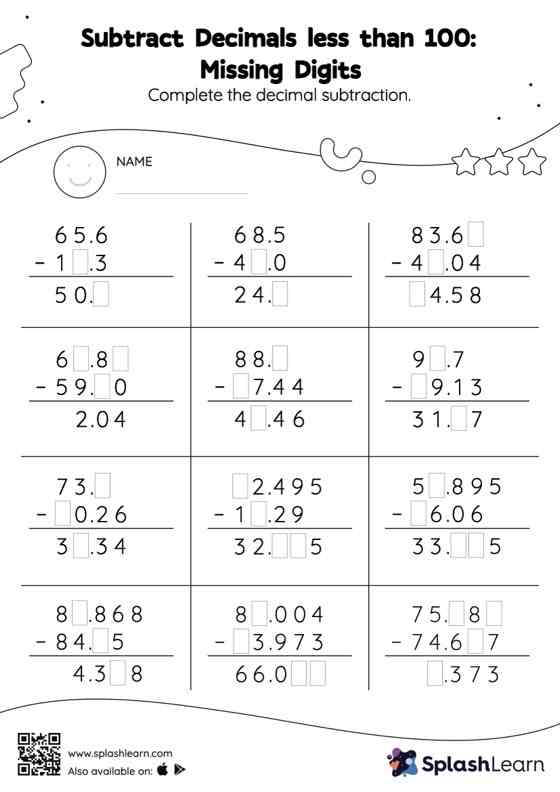# Subtract Decimals less than 100: Missing Digits Worksheet

Home > Subtract Decimals less than 100: Missing DigitsStudents align the decimal points and use zero as space holders to subtract decimals. Then they use the relationship between addition and subtraction to find the missing number in subtract decimals less than 100 worksheet. As the worksheet uses the column method, it is helpful in getting students toward higher accuracy, especially with bigger numbers and in scenarios where regrouping is required.